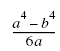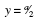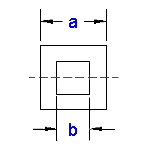Area Moment of Inertia Section Properties of Square
@ Center Tube Calculator

Area Moment of Inertia Section Properties of Square Tube at Center Calculator and Equations

This engineering calculator will determine the section modulus and Area Moment of Inertia Section Propertiesfor the given cross-section. This engineering data is often used in the design of structural beams or structural flexural members. There are two types of section moduli, the elastic section modulus (S) and the plastic section modulus (Z).

 Description Equation Area Moment of Inertia Section Properties = ISection Modulus = Z = I/yRadius of GyrationA = areay = distance from axis to extreme fiberArea Moment of Inertia Section Properties of Square Tube at Center Calculator Inputs: Inch (in.) Metric (mm) "a"  (Exterior Side) = "b"  (Interior Side) = Properties: Area Moment of Inertia Section Properties (Units4) = Section Modulus (Units3) = Radius of Gyration (Units) = Extreme Point (Units) = Area (Units2) =© Copyright 2000 - 2019, by Engineers Edge, LLC www.engineersedge.comMembership Register | LoginHomeEngineering Book StoreEngineering ForumExcel App. DownloadsOnline Books & ManualsEngineering NewsEngineering VideosEngineering CalculatorsEngineering ToolboxGD&T Training Geometric Dimensioning TolerancingDFM DFA TrainingTraining Online EngineeringAdvertising CenterCopyright Notice